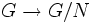Quotient map

Contents

VIEW RELATED: Analogues of this | Variations of this | Opposites of this |[SHOW MORE]

Definition

Symbol-free definition

Given a group and a normal subgroup, the quotient map corresponding to that normal subgroup is the map from the group to the coset space (that is, the set of cosets) of this normal subgroup.

Since the equivalence relation induced by being in the same coset of the normal subgroup is a congruence, the coset space can actually be equipped with a canonical group structure, and the quotient map then becomes a surjective group homomorphism.

The coset space with this group structure is also termed the quotient group.

Definition with symbols

Let$G$ be a group and$N$ be a normal subgroup. Consider the map$\varphi:G \to G/N$ which sends$g \in G$ to the coset$gN$. This map is termed the quotient map.

The equivalence relation induced by the cosets of a normal subgroup is a congruence, viz.:

•$aN = bN, cN = dN \implies acN = bdN$
•$aN = bN \implies a^{-1}N = b^{-1}N$

Thus, we can define a group structure on the coset space by setting$(aN)(bN) = abN$ (this is well-defined and independent of the choice of coset representatives precisely because of the above fact).

Note that the quotient map is a surjective homomorphism whose kernel is the given normal subgroup.

The group$G/N$ is also termed the quotient group of$N$ via this quotient map.

Related facts

• Normal subgroup equals kernel of homomorphism: The kernel of any homomorphism is a normal subgroup. Conversely, any normal subgroup is a kernel of a homomorphism, namely the quotient map for that normal subgroup.
• First isomorphism theorem: This states that for any homomorphism of groups$\varphi:G \to H$ with kernel$N$, the map from$G$, restricted to the image, is equivalent to the quotient map$G \to G/N$
• Second isomorphism theorem
• Third isomorphism theorem
• Fourth isomorphism theorem